## 有机化学

### 有机化学入门章节

• $$π$$-$$π$$共轭体系
• $$p$$-$$π$$共轭体系
• 超共轭体系：
• $$σ$$-$$π$$共轭
• $$σ$$-$$p$$共轭

(1) 诱导效应和共轭效应

SN1 和SN2  补充

## 原子结构和分子结构

### 范德华力

The van der Waals force is a distance-dependent interaction between atoms or molecules. Unlike ionic or covalent bonds, these attractions do not result from a chemical electronic bond; they are comparatively weak and therefore more susceptible to disturbance. The van der Waals force quickly vanishes at longer distances between interacting molecules.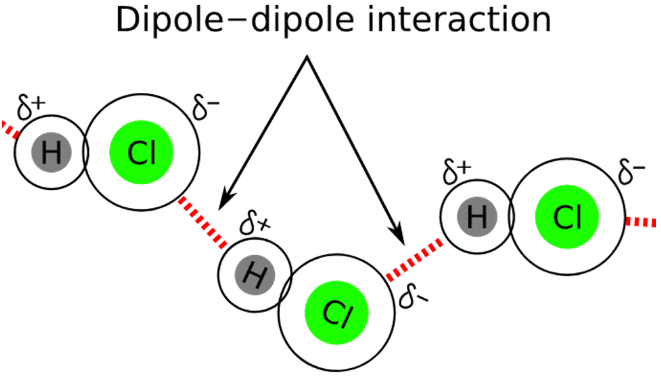Keesom Forces: Keesom forces are the temperature dependent and very weak van der Waals forces that originate between permanent dipoles. As, molecules rotate constantly and it is generally assumed that they cannot be locked in one place, but at some points, they do get locked. These interactions arise at those moments and are seen in all quadrupoles and multipoles. Keesom forces are named after William Hendrik Keesom and arise due to interactions between permanent polar structures.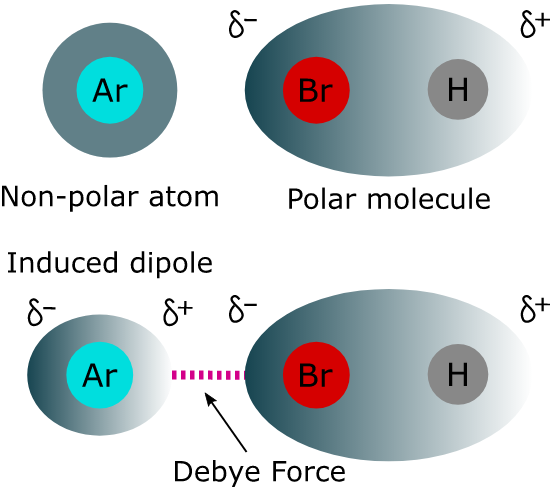Debye force is only attractive and does not disappear at higher temperatures. It cannot occur between atoms, unlike the London dispersion force.

(1) Argon molecule experiences dipolar characteristics when attracted by the hydrogen atom of HCl or repelled by the chlorine atom of HCl.
(2) Polar molecules such as water, create dipoles in neutral molecules like oxygen, etc.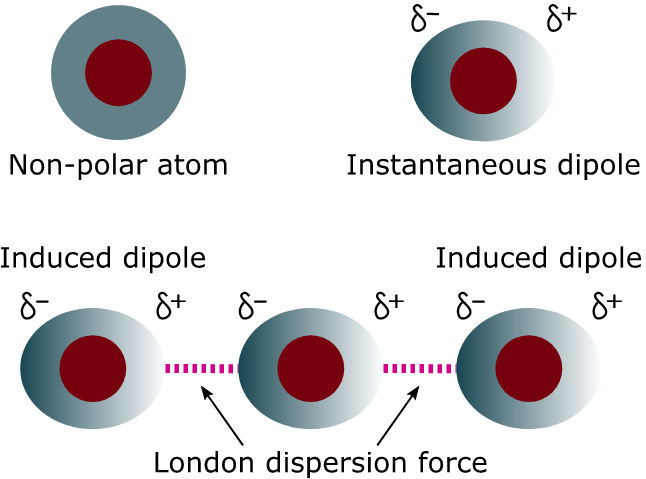伦敦色散力(London Dispersive Forces): Also known as the “temporary dipole-induced dipole”, “instantaneous dipole-induced dipoles”, or “fluctuating dipole-induced dipole". The electron distribution around an atom or molecule undergoes fluctuations in time. These fluctuations create instantaneous electric fields which are felt by other nearby atoms and molecules, which in turn adjust the spatial distribution of their own electrons. The net effect is that the fluctuations in electron positions in one atom induce a corresponding redistribution of electrons in other atoms, such that the electron motions become correlated. 总而言之就是形成instantaneous dipoles attract each other. 更深层次的原因需要用量子力学来解释。

• Forces are attractive
• Forces are present between all atoms – polar and nonpolar
• Larger atoms display stronger forces than smaller ones, i.e., the strength increases down a particular group in the periodic table
• Easily polarizable atoms and molecules have stronger forces than harder polarizable ones
• Cylindrically shaped molecules allow more molecules to come close to one another than spherical ones, thereby increasing their strength

wiki的描述：科学家观察到在高压低温下，即使是非极性分子也能被液化或固化，弗里兹·伦敦认为非极性分子间必然有吸引力存在，否则在任何条件下非极性分子在任何条件下应该都是气体，此吸引力即为伦敦色散力。 其能量很小，存在所有分子之间。非极性分子内的电子云运动会造成瞬间分布不均匀，产生瞬间偶极，此瞬间偶极又会使附近的非极性分子产生暂时的诱发偶极现象并且是在低概率的可能性中发生的，使非极性分子互相吸引，此种作用力又称瞬间偶极-诱发偶极力。

   Dipole-dipole Forces London Dispersion Forces Definition Occurs between two polar molecules Occurs due to the presence of temporary dipole moments caused by moving electrons Strength Stronger than dispersion forces Weaker than dipole forces Polarity Permanent Temporary Examples HCl, H2O, and acetone He, Cl2, and CCl4

### 多电子原子轨道能级

(1) Pauling近似能级图(高中老师讲的部分)

“能级分裂”：主量子数$$n$$相同，角量子数$$l$$不同的能级，能量随$$l$$的增大而升高。
“能级交错”：当主量子数$$n$$和角量子数$$l$$均不同时，出现能级交错现象，比如$$E_{4 s}<E_{3 d}<E_{4 p} \cdots$$。

(2) Cotton能级图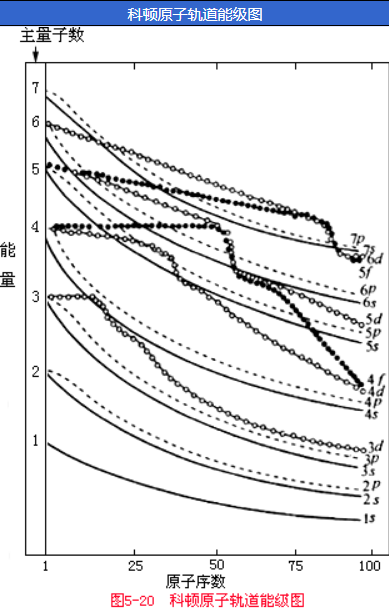• 反映出主量子数相同的氢原子轨道的简并性。对氢元素来说，其主量子数相同的各轨道全处于同一能量点上，即不存在能级分裂现象。
• 反映出原子轨道的能量随着原子序数的增大而降低。
• 反映出随着原子序数的增大，原子轨道能级下降幅度不同，因此能级曲线产生了相交现象。例如$$3d$$和$$4s$$轨道的能量高低关系：K，Ca的$$E_{3 d}>E_{4 s}$$；而原子序数较小或较大时，$$E_{3 d}<E_{4 s}$$。

(3) 屏蔽效应

He原子核内含有2个质子，描述He原子的能量状态，不仅要考虑原子核对外层电子的吸引能和外层电子自身的动能，还要考虑两个电子之间的排斥能。He原子的薛定谔方程难解的根源是排斥能的存在，从而难以精确地说明一个电子对另一个电子的影响。解决的办法就是用近似处理法。我们可以认为，He原子中的一个电子对另一个电子产生了电荷屏蔽，即削弱了核电荷对该电子的吸引力。于是有效核电荷数$$Z^{*}=2-\sigma$$，其中$$\sigma$$为核电荷的减小值，称为屏蔽常数。

(4) 钻穿效应

### 核外电子排布

(1) 基态原子的核外电子排布原则

• 能量最低原理，电子在原子轨道中的排布，要尽可能使整个原子系统能量最低。
• Pauli不相容原理，同一原子轨道最多容纳两个自旋方式相反的电子，或者说同一原子中不能有一组四个量子数完全相同的电子。
• Hund规则，在相同$$n$$和相同$$l$$的轨道上分布的电子，将尽可能分占$$m$$不同的轨道，且自旋平行。

(2) 基态原子的核外电子排布

Cr和Cu原子的原子序数分别为24和29，其原子的电子排布分别为$$[\mathrm{Ar}] 3 \mathrm{~d}^{5} 4 \mathrm{~s}^{3}$$和$$[\mathrm{Ar}] 3 d^{10} 4 s^{1}$$，而不是$$[\mathrm{Ar}] 3 d^{4} 4 s^{2}$$和$$[\mathrm{Ar}] 3 \mathrm{~d}^{9} 4 \mathrm{~s}^{2}$$，因为半充满和全充满结构是能量较低的稳定结构。

### 元素性质的周期性

(1) 原子半径

• 金属半径：金属单质晶体中，两个最近邻金属原子核间距的一半。
• 共价半径：同种元素的两个原子以共价单键结合时，其核间距的一半。
• 范德华尔斯半径：分子晶体是以范德华尔斯力结合的，比如稀有气体形成的单原子分子晶体中，两个同种原子核间距的一半。

•  随着核电荷的增加，原子核对外层电子的吸引力增强，使原子半径逐渐变小；
• 随着核外电子数的增加，电子间的斥力增强，使原子半径变大。
由于前者的作用比后者更显著，即增加的电子不足以完全屏蔽所增加的核电荷，所以从左向右，有效核电荷逐渐增大，原子半径逐渐变小。

(2) 电离能 (Ionization Energy)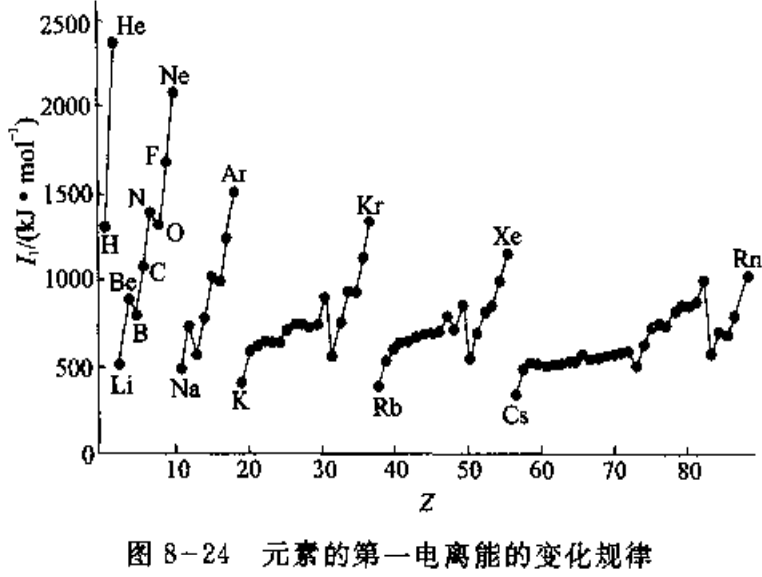• 同一周期，从碱金属到卤素，元素的有效核电荷逐个增加，原子半径逐个减小，电离能逐个增大。第一主族的最小，稀有气体的最大。
• 长周期的中部元素(即过渡元素)，由于电子加到次外层(ns，(n-1)d，np或者ns，(n-2)f，(n-1)d，np)，有效核电荷增加不多，原子半径减小缓慢，电离能仅略有增加。
• N，P和As等的电离能较大，Be和Mg的电离能也较大，均比它们后面的元素的电离能大，这是半满和全满状态，比较稳定，失去电子相对较难。
• 同一族从上到下，最外层电子书相同，有效核电荷增加不多，原子半径的增大成为主要因素，致使核对外层电子的引力依次减弱，电子逐渐易于失去，电离能依次减小。
• 电离势(ionization potential)和电离能是同一个意思，但是前者是an older and obsolete term。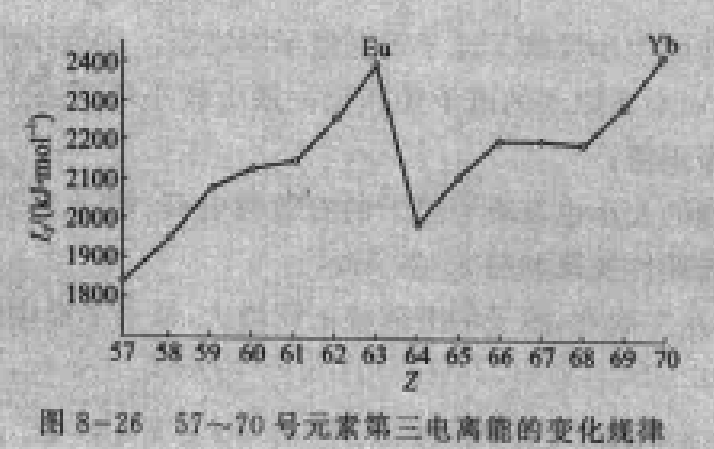(3) 电子亲和能(Electron Affinity)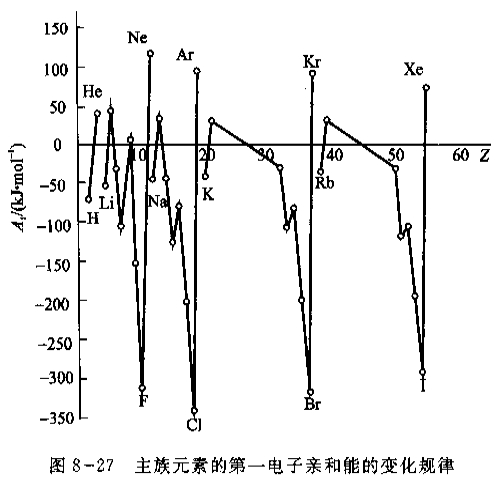• 电子亲和能的大小反映了原子得到电子的难易。非金属原子的第一电子亲和能总是负值，而金属原子的电子亲和能一般为较小负值或正值。稀有气体的电子亲和能均为正值。
• 电子亲和能的大小也取决于原子的有效核电荷、原子半径和原子的电子层结构。
• 同一周期，从左到右，原子的有效核电荷增大，原子半径逐渐减小，同时由于最外层电子数逐渐增多，趋向于结合电子形成8电子稳定结构。元素的电子亲和能的负值在增大。卤素的电子亲和能呈现最大负值。
• 碱土金属因为半径大，且有$$n \mathrm{~s}^{2}$$电子层结构难以结合电子，电子亲和能为正值。
• 稀有气体具有8电子稳定构型，更难以结合电子，因此电子亲和能为最大正值。
• N原子比较特殊，电子亲和能是正值，是p区元素中除稀有气体外唯一的正值，这是由于它具有半满p亚层稳定电子构型，加之原子半径小，电子间斥力大，得电子困难。
• Cl原子的电子亲和能的负值最大，而不是F原子，这可能是由于F原子的半径小，进入的电子受到原有电子较强的排斥，用于克服电子排斥所消耗的能量相对多一些。

(1) 注意国内外对电子亲和能的正负值是相反的，参考这里wiki上的正负值和上图相反，和IUPAC Gold Book一致。大多数情况下体系放出能量应该取负值，但是习惯上(IUPAC)电子亲和能正好相反，取正值说明体系放出能量。
(2)
Eu2+-Tb3+的pair的基态比Eu3+-Tb2+的基态低。

(4) 电负性

电负性是原子在分子中吸引电子的能力，不是一个孤立原子的性质，而是在周围原子影响下的分子中原子的性质。为了比较不同原子的电负性，Pauling最早建立了电负性标度，他把氢的电负性指定为2.2的基础上，基于相关分子的键能数据，与H的电负性对比，得到其他元素的电负性值，因此个元素原子的电负性是相对数值。

Pauling电负性标度$$\chi_{\mathrm{P}}$$是目前广泛使用的一种，电负性标度有多种，数据各有不同，但在周期系中电负性变化规律是一致的。电负性可以综合衡量各种元素的金属性和非金属性。Pauling电负性标度中，金属元素的电负性一般在2.0以下，之上为非金属元素。同一周期，从左到右电负性依次增大，元素的非金属性增强，金属性减弱；同一主族，从上到下，电负性依次变小，元素的非金属性减弱，金属性增强。过渡元素的电负性递变不明显，它们都是金属，但金属性都不及第一和第二主族元素。

(1)  中山大学无机化学复习
(2) 核外电子排布和元素周期律

### Lewis理论

(1) 存在电荷排斥的两个电子为何能以共用电子对的形式将两个原子结合在一起，Lewis理论无法给以说明。
(2) Lewis结构式并不代表分子形状，而仅仅代表成键方式和键的数目。
(3) 存在相当数量不符合“八隅体规则”的例子，比如BF3，PCl5和SF6这些稳定分子。

(1) BF3， 三氟化硼气体刺激性强，加热或与湿空气接触会分解形成有毒和腐蚀性的氟化氢气体，腐蚀眼睛、呼吸道和皮肤。高纯三氟化硼三氟化硼常用于半导体集成电路生产的掺杂、离子注入等工艺中。
(2) PCl5可用于六氟磷酸锂(电解液的重要组成)的制备
$$\quad \quad \quad \quad \quad \mathrm{PCl}_{5}+6 \mathrm{LiF} \rightarrow \mathrm{LiPF}_{6}+5 \mathrm{LiCl}$$
(2-a) 湿法：该方法是将锂盐溶于无水氢氟酸中形成LiF·HF溶液，然后通入PF5气体进行反应生产六氟磷酸锂结晶。经分离，干燥得到产品。
(2-b) 干法：该方法是将LiF用无水HF处理，形成多孔LiF，然后通入PF5气体进行反应，从而得到产品。
(3) SF6，根据VSEPR理论它是nonploar分子，the fluorine atom is higher electronegative than sulfur atom due to which the fluorine atom attracts the bonded pair of electron slightly towards itself. As a result, the S-F bond becomes polar and results in a dipole moment but due to symmetry, the dipoles get canceled out with each other making the SF6 a nonpolar molecule.[reference]

SF6 is used in the electrical industry as a gaseous dielectric medium for high-voltage sulfur hexafluoride circuit breakers, switchgear, and other electrical equipment, often replacing oil-filled circuit breakers (OCBs) that can contain harmful polychlorinated biphenyls (PCBs). [wiki]

### 价键理论

(1) 原子中自旋方向相反的未成对电子相互接近时，可以相互配对形成稳定的化学键。一个原子有几个未成对电子，就可以与几个自旋相反的未成对电子配对成键。
(2) 形成共价键时，成键电子的原子轨道必须在对称性一致的前提下发生重叠，原子轨道的重叠程度越大，两核间电子的概率密度就越大，形成的共价键就越稳定。

(1) 共价键具有饱和性。
(2) 共价键具有方向性。除了s轨道外，p、d和f轨道在空间都有一定的伸展方向，成键的时候只有沿着一定的方向取向，才能满足最大重叠原则。

$$σ$$键：由两个相同或不相同的原子轨道沿轨道对称轴方向相互重叠而形成的共价键，叫做$$σ$$键。共价单键是$$σ$$键，共价双键有一个$$σ$$键，$$π$$键，共价三键由一个$$σ$$键，两个$$π$$键组成。
(1) $$σ$$键有方向性，两个成键原子必须沿着对称轴方向接近，才能达到最大重叠。
(2) 成键电子云沿键轴对称分布，两端的原子可以沿轴自由旋转而不改变电子云密度的分布。
(3) $$σ$$键是头碰头的重叠，与其它键相比，重叠程度大，键能大，因此，化学性质稳定

$$π$$：在化学上是共价键的一种。当两个电子轨道的突出部分发生重叠时产生。名字中的希腊字母$$π$$代表了p轨道，因为$$π$$键的轨道对称性与轨道相同。p轨道通常参与形成$$π$$键，然而，d轨道同样能参与形成。 $$π$$键通常比$$σ$$键弱，因为它的电子云距离带正电的原子核的距离更远，需要更多的能量。由一个$$σ$$键和一个$$π$$键组成的C=C双键的键能小于C-C单键的两倍,表明$$π$$键所增加的稳定性小于$$σ$$键的稳定性。量子力学的观点认为，键的强度很弱主要是因为平行的p轨道间重叠不足。这与$$σ$$键形成对比，$$σ$$键直接在键合原子的核之间形成键合轨道，使得有更大的重叠区,所以$$σ$$键的强度也更强。

The possible coordination geometries around copper in Cu(NH3)42+ span a continuum from square planar to tetrahedral. Square planar coordination produces a much larger gap between the d orbitals of the complex than does tetrahedral coordination. The color of the complex arises from excitation of an electron from one d orbital to a higher energy d orbital. Given this fact, and the fact that most d-d electronic transitions occur in the visible region, which coordination geometry (tetrahedral or square planar) do you think is more likely for Cu(NH3)42+? Provide a brief rationale for your answer. Note: the color of the complex arises from light that is not absorbed.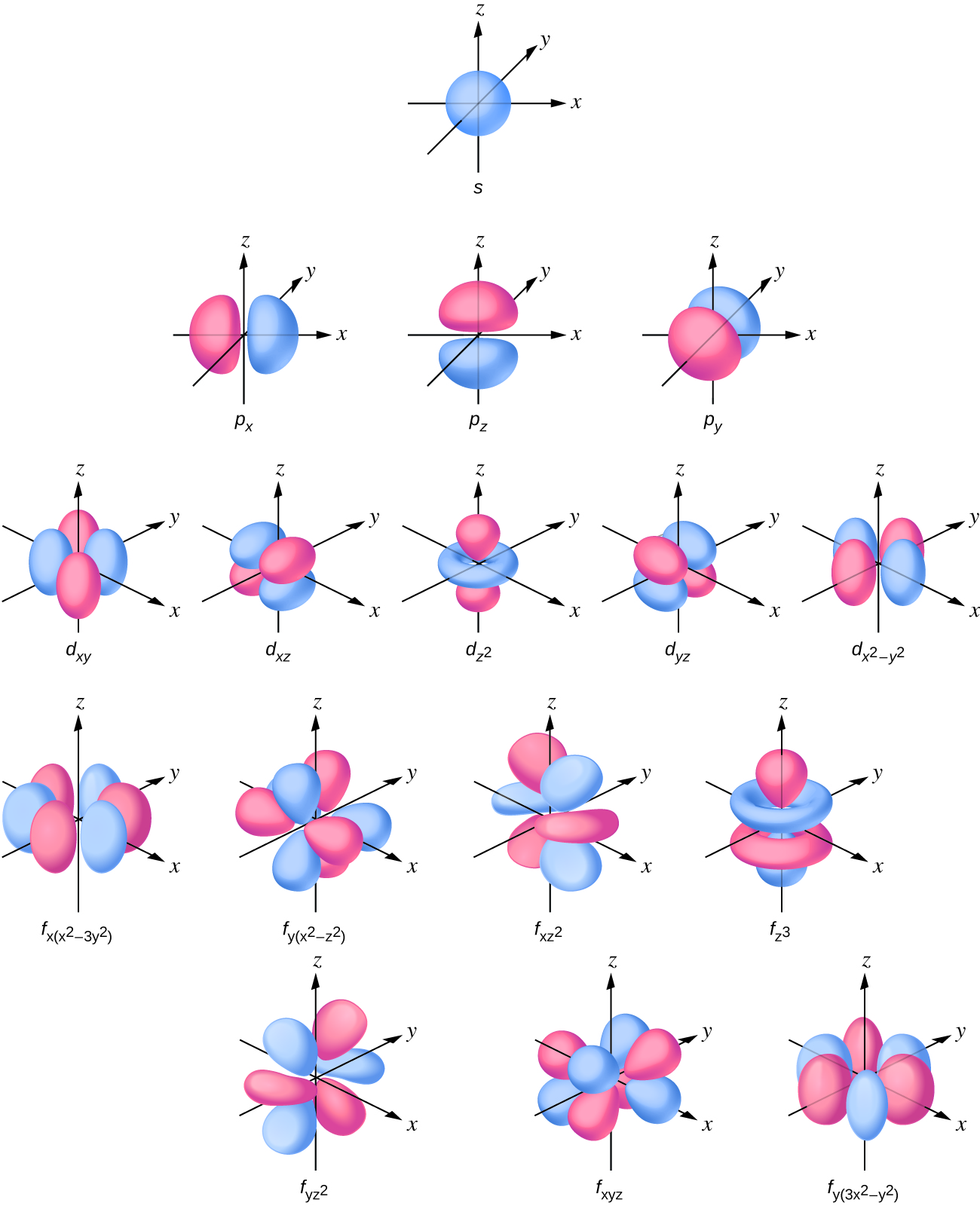### 分子轨道理论

Lewis的电子对成键理论直观、简明，价键理论教好地说明了共价键的行程，为引入量子力写处理分子结构奠定了基础。但是该理论把行程共价键的电子只定域在两个相邻原子之间，没有考虑整个分子的情况，因此不能解释某些分子的性质：
(1) O2分子有顺磁性，表明含有未成对电子，但是其路易斯结构式电子都成对了；
(2) H2+和He2+的形成；
(3) B2H6等缺电子化合物，价键理论无法解释；
(4) 无法解释物质的颜色。

(1) 在分子中的电子不局限在某个原子轨道上运动，而是在分子轨道上运动。分子中每个电子的运动状态可以用波函数$$\psi$$来描述，此$$\psi$$称为分子轨道
(2) 分子轨道可以由组成分子的原子的原子轨道线性组合而成。例如，原子A和B的原子轨道波函数分别为$$\psi_A$$和$$\psi_B$$，它们线性组合成两个分子轨道：\begin{aligned} \phi_{1} &=C_{1} \psi_{\text {A }}+C_{2} \psi_{\mathrm{B}} \\ \phi_{2} &=C_{1} \psi_{\mathrm{A}}-C_{2} \psi_{\mathrm{B}} \end{aligned}(a) 组合形成的分子轨道与组合前的原子轨道数目相等，但是能量不同。
(b) $$\phi_{1}$$是能量低于原子轨道的成键分子轨道，是原子轨道同号重叠(波函数相加)形成的，电子出现在核间区域概率密度大，对两个核产生强烈的吸引作用，形成的键强度大。反之$$\phi_{2}$$，波函数异号，电子密度减小，不利于成键。
(c) 节面就是波函数位相正负号发生改变的地方(要么平行于键轴，要么垂直于键轴，该处的电子云密度为零)。$$π$$分子轨道有通过键轴(原子核的连线)的节面，而$$σ$$分子轨道没有通过键轴的节面。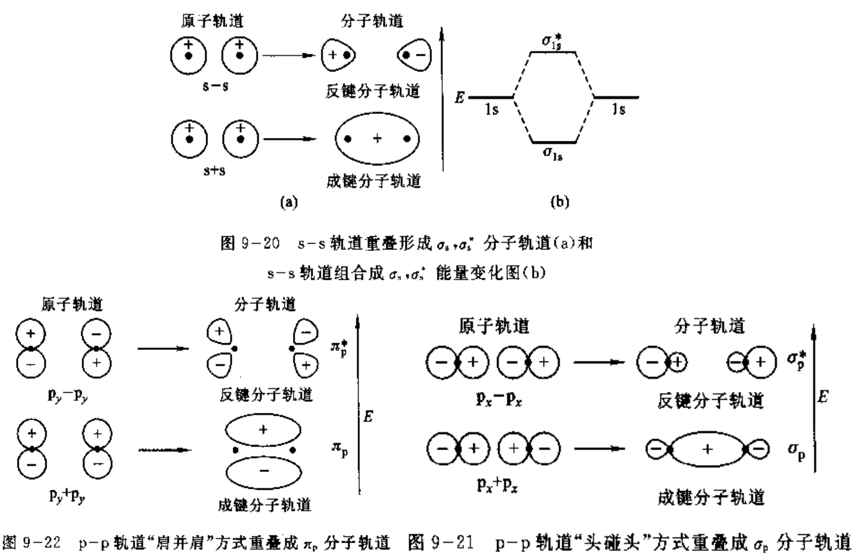(1) 原子轨道倾向于与能量相近的其他原子轨道结合；
(2) 两个原子轨道的重合部分越多，形成的成键分子轨道能量越低，反键分子轨道能量越高，即分裂能越大；
(3) 每一个分子轨道最多可以容纳一对自旋方向相反的电子；
(4) 如有两个能量相同的分子轨道，电子的排布遵循洪特规则(即如有两个电子，则各自分布在两个轨道中，且自旋方向相同)。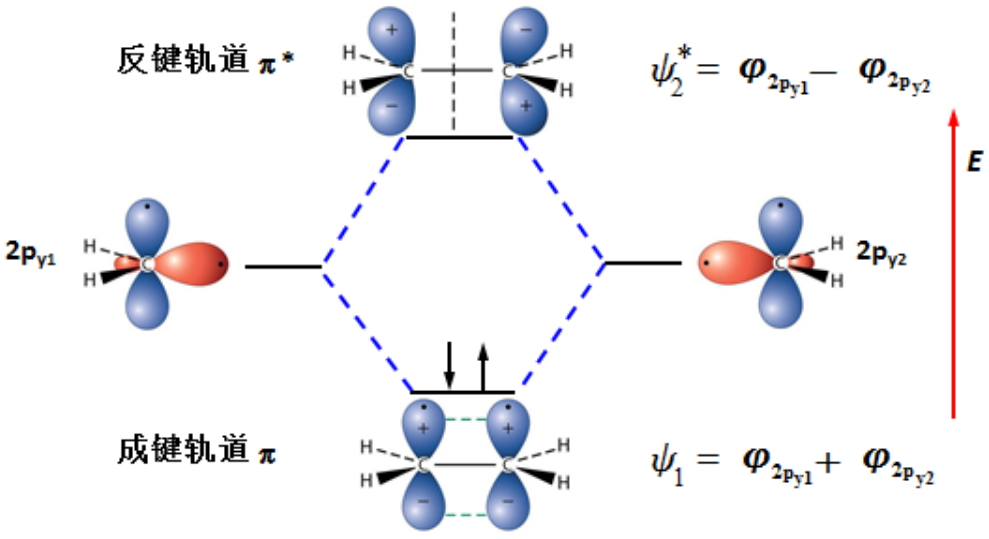DS

## 酸碱理论

1.负离子：卤离子、氢氧根离子、烷氧基离子、烯烃、芳香化合物
2.带有孤电子对的化合物：氨、胺、醇、醚、硫醇、二氧化碳
在有机化学中Lewis碱是亲核试剂。

(1) 孤对电子亲核试剂；(2) $$σ$$键亲核试剂；(3) $$π$$键亲核试剂。

：是指那些具有较高电荷密度、较小半径的粒子(离子、原子、分子)，即电荷密度与粒子半径的比值较大。极化性(polarizable)较低，但极性较大。
：是指那些具有较低电荷密度和较大半径的粒子。极化性较高，但极性较小。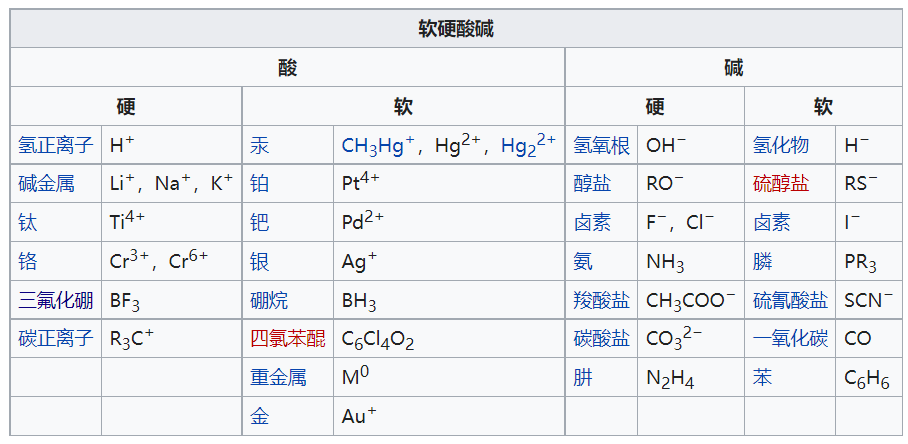## 配位化合物

(1) 一是中心原子或离子，它必须有能接受电子的空轨道；
(2) 二是有配位体，而且配位体中要键合的原子能提供孤对电子。

## 化学动力学

Fundamental concepts in chemical kinetics-ChemTexts

### 化学平衡

Some Considerations on the Fundamentals of Chemical Kinetics: Steady State, Quasi-Equilibrium, and Transition State Theory

$$k=A e^{-E_{a} / R T}$$ 或 $$\ln k=-\displaystyle\frac{E_{a}}{R T}+\ln A$$

### 过渡状态理论

(1) 通过研究建立在势能平面上的马鞍点处的活化络合物来研究反应速率。而该活化络合物是怎样形成的细节并不重要。
(2) 活化络合物与反应物分子处于特定的平衡(准平衡)。
(3) 活化络合物可以转化成不同的产物，而这种转化速率可以用动力学理论计算。

Eyring方程：补充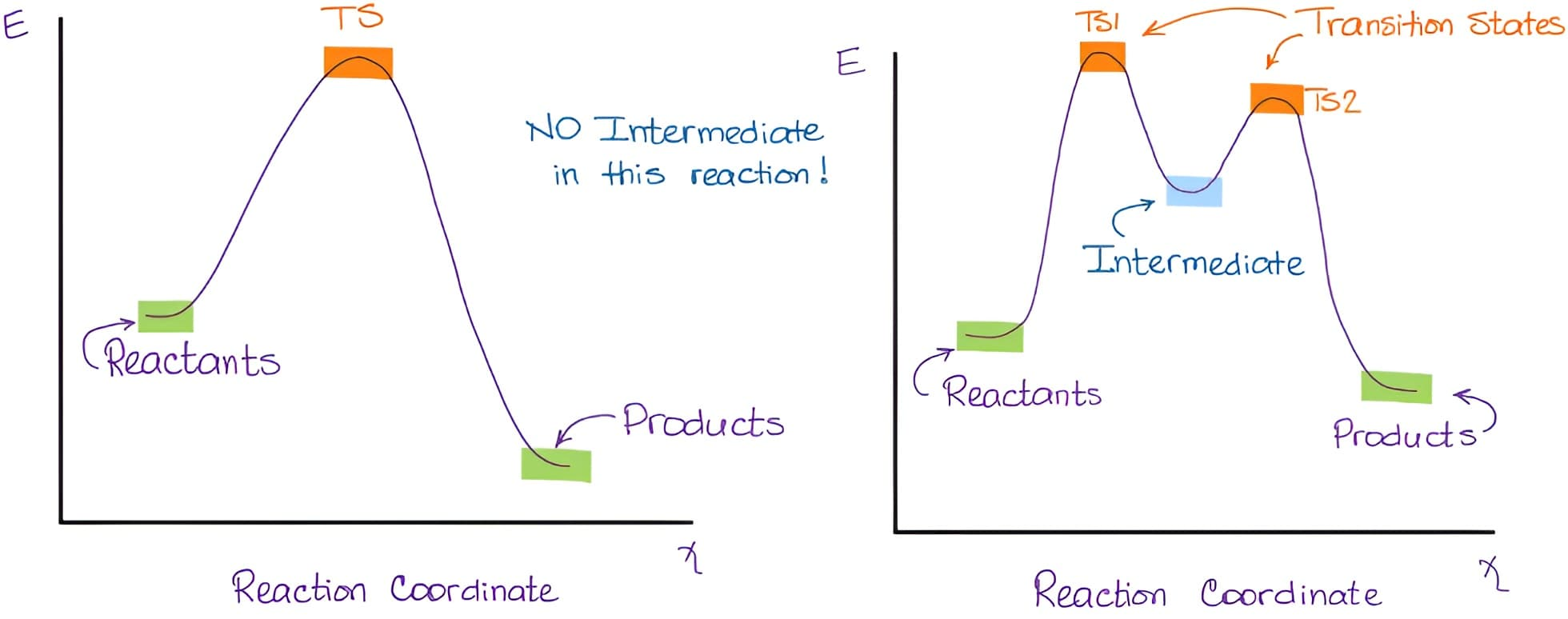### 微分方程

• Steady state is established in kinetically controlled processes;
• A → B → C   (单位时间内由A生成的B的数量等于单位时间内由于生成C而消耗的B的数量，即B的浓度不随时间变化)
In open systems, equilibrium can not be achieved but the steady-state can be achieved easily when incoming or outgoing heat or mass are equal
• There may be a transfer of heat due to an open system.
• The system is not at equilibrium which states that the entropy is increasing with time

• Equilibrium is achieved in thermodynamically controlled processes.
• A ⇋ B  (单位时间内由A生成的B的数量等于单位时间内由于B生成A的数量，即A、B的浓度不随时间变化)
In equilibrium, A can be changed into B and B can be changed into A, but net concentration remains constant
• In equilibrium, no net heat transfer due to a closed system.
• An equilibrium is established in a closed system.
注：百度百科的介绍来看应该是孤立系统而不是封闭系统。平衡态(equilibrium state)是指在没有外界影响条件下热力学系统 的各部分宏观性质在长时间里不发生变化的状态。这里所说的没有外界影响，是指系统与外界没有相互作用，既无物质交换，又无能量传递(做功和传热)，即系统是孤立系。
• The entropy remains constant in equilibrium.
• In equilibrium, no net heat transfer due to a closed system.

## 化学热力学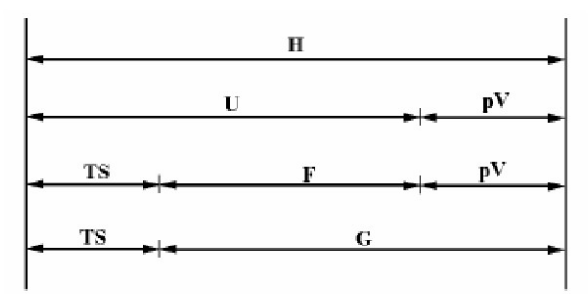(1) 内能：内能是指系统所含有的能量，但不包含因外部力场而产生的系统整体之动能与势能。内能是一系统内的状态函数，因为其值仅取决于该系统的目前状态，而与达到此一状态所采之途径或过程无关。虽然内能是个宏观物理量，内能也可在微观层面上由两个假设的量来解释。一个是系统内粒子的微观运动(平移、旋转、振动)所产生的微观动能。另一个是与粒子间的化学键及组成物质的静止质量能量等微观力有关之势能。就热力学及工程学上的实际用途来看，一般很少需要考虑一个系统的所有内含能量，如质量所含有的等价能量。一般而言，只有与研究的系统及程序有关的部分才会被包含进来。热力学一般只在意内能的“变化量”。

(2) 吉布斯自由能(也叫自由焓)：在等温等压状况(有的写恒温恒压，状态函数，强度量，不影响)下，一个热力过程具有自发性的必需条件为，吉布斯能随着过程的演化而减小。这意味着，平衡系统的吉布斯能是最小值；在平衡点，吉布斯能对于其它自变量的导数为零。

$$G = H - TS$$, 热力学自由能(包括吉布斯自由能和亥姆霍兹自由能)是指一个热力学系统的能量中可以用来对外做功的部分。简单的来说，自由能越大，这个系统的能量变成的另一种能量选择也就越多。所以说，其他条件相等的情况下，熵越大，自由能就越小，系统能量中能用来做功的部分越少，这个系统的“变数”越少。(参考知乎)

(3) 亥姆霍兹自由能：在物理学中也常直接简称为自由能，自由能可以被理解成是系统内能的一部分，这部分在可逆等温过程中被转化成功。$$F = U -TS$$或$$A = U -TS$$

(4) ：借用知乎1知乎2答案可以形象理解，$$H = U+PV$$。把一个物体凭空变出来，除了需要增加该物体具有的内能$$U$$，还要排开该物体所占体积的空气，这个过程会做功$$PV$$，因此，焓可以理解为凭空变出一个物体需要的总能量。严格来说，焓并没有物理意义。

(5) 大势能：巨热力学势，也称作朗道自由能，是统计力学中使用的一个量，特别是在开放系统的不可逆过程里使用。在统计力学中，它作为巨正则系综的特性函数出现。

(1) 体积功和非体积功：热力学系统只因膨胀对外做功(或外界做功)称为体积功，而非体积功是诸如电/磁介质极化、表面张力等等做的功。(电功、表面功、光、轴功等)

(2) 自由膨胀：free expansion是一种不可逆的热力学过程，过程中气体在一个绝热的系统内膨胀(其实是向真空膨胀)，外界和系统之间没有功和热的交换。 真实气体在自由膨胀时会有温度的变化。若是理想气体，过程前后的温度不会变化，内能只和温度有关。

(3) 耗散过程：通过摩擦使功变为热的效应(摩阻、电阻、非弹性变性、磁阻等)。比如活塞中气体膨胀，如果活塞存在摩擦，那么系统对外界做的功大于外界得到的功。

(4) 准静态过程：在热力学里，准静态过程是系统总和环境处于热平衡的过程。现实中准静态过程不存在，但“足够(sufficiently)缓慢”的过程可视为一良好的近似。准静态过程可以可逆，亦可不可逆。当过程中熵增加时，则为不可逆。反之，任何可逆过程都必为准静态。但应注意若两物体间交换速率为一绝热的隔板所控制时，不论过程发生得如何缓慢，两个物体的状态都绝不会无限地趋近平衡态，因为热平衡需要两个物体具有绝对相同的温度(不太懂)。在有些文献中，对于准静态过程及可逆过程是有些模糊不明的，且有时它们会被当做同义词。准静态过程，既是平衡，又有变化。

(5) 可逆过程：准静态过程 + 无耗散效应 = 可逆过程

• 准静态过程是实际过程的理想化过程， 但并非最优过程，可逆过程是最优过程。
• 可逆过程的功与热完全可用系统内工质的状态参数表达，可不考虑系统与外界的复杂关系，易分析。
• 实际过程不是可逆过程，但为了研究方便，先按理想情况(可逆过程)处理， 用系统参数加以分析，然后考虑不可逆 因素加以修正。

• 可逆膨胀和可逆压缩：力学平衡。(从同一状态出发经过不同绝热过程到达相同的体积(或相同的压力)，则其中可逆过程的功最大)
• 可逆传热：热平衡
• 可逆相变：相平衡
• 可逆化学反应：A ＋ B ⇔ C

(6) 不可逆过程：实际过程进行有方向性，满足能量守恒，但是不能返回原状态。常见的不可逆过程有不等温传热、节流过程、自由膨胀、混合过程。具体来说，在不考虑耗散下，高压气体通过活塞向外等温膨胀，从状态A到状态B膨胀对外做的功为W1，然后外界压缩气体使之回到A状态，外界对系统做的功为W2，显然W2大于W1，即在环境中留下了影响。

(7) 等熵过程：

(8) 热力循环的评价指标：参考李永乐老师9分钟讲解热机原理和卡诺定理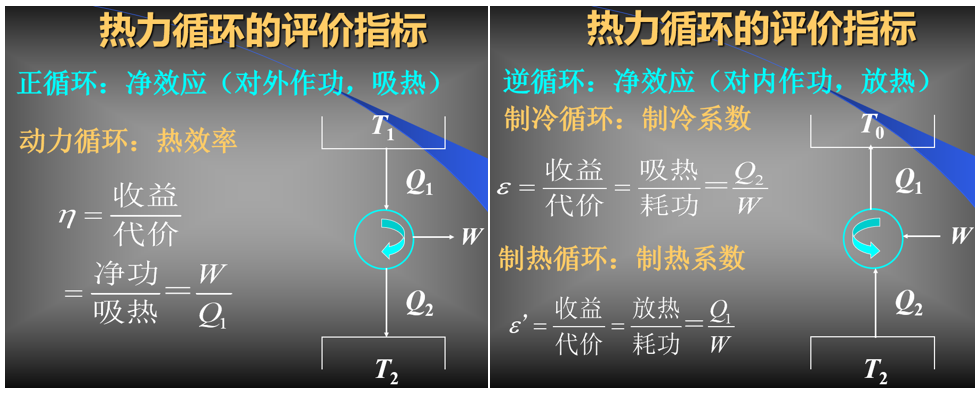(9) 热容

(10) 节流过程与焦耳-汤姆孙(Joule-Thomson)实验

(11) 自发过程

(a) 自发过程单向地朝着平衡。
(b) 自发过程都有作功本领。
(c) 自发过程都是不可逆的。

(12) 熵：很多情况下，近似认为反应的熵变基本不随温度而变。

(a) 数学上，第零定律表示这是一种等价关系。
(b) 第零定律说明任何两个系统的热平衡关系都是等价的，而经常被认为可于建立一个温度函数；更随便的说法是可以制造温度计。

(a) 物体内能的增加等于物体吸收的热量和对物体所作的功的总和。
(b) 系统在绝热状态时，功只取决于系统初始状态和结束状态的能量，和过程无关。
(c) 孤立系统的能量永远守恒。
(d) 系统经过绝热循环，其所做的功为零，因此第一类永动机是不可能的(即不消耗能量做功的机械)。
(e) 两个系统相互作用时，功具有唯一的数值，可以为正、负或零。

(a) 一个是做功与传热在改变热力学平衡态的效果上具有等价性；
(b) 二是改变热力学平衡态的方法只有做功与传热两种途径。

(a) 克劳修斯表述：不可能把热量从低温物体传递到高温物体而不产生其他影响。

(b) 开尔文表述：不可能从单一热源吸收能量，使之完全变为有用功而不产生其他影响。

1.开尔文表述和克劳修斯表述是等价的

2. 网友也说了一种理解方式“其实这还是力学,当两个速度不一的分子互相撞击时,速度快的会变慢,速度慢的会变快”。

3. 热力学第二定律代表了自然界的前进方向，有些事情是可以发生的，有些事情虽然满足能量守恒，但是不能发生。能量是在转化和守恒过程中的，但是能量的品质是有区别的，高温热源向低温热源传递热量，二者温度相等，就不能再做功了，我们就称之为能量的品质降低了。

4. 热机的做法都是有两个热源，低温热源，高温热源，从高温热源吸收一部分热，这部分热一部分给了低温热源，剩下的部分才是用来对外做功。内燃机就是一个例子，如果周围的空气和内燃机的温度是一样的，那么就无法对外做功，对外做功的前提是有温度差。

5.热功转换的不等价性，功可以无代价地全部变为热，但热全部变功必须付出代价(系统和环境)，若不付代价只能部分变功。

6. 参考资料：李永乐-热力学第二定律是什么？“麦克斯韦妖”是什么鬼？### 化学热力学的应用实例

1.通过热力学参量来计算结合能或晶格能。例如，MgO的晶格能可以通过如下的循环来求得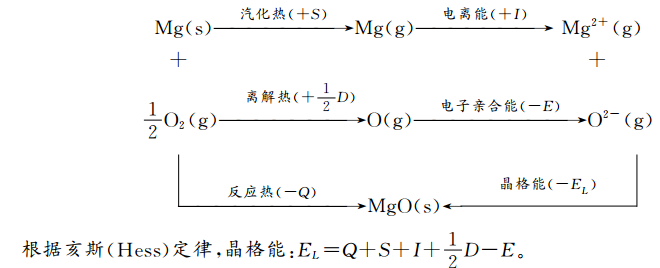等式右边各参量都可测量，则晶格能可由实验数据计算出来。

2.计算热缺陷浓度

$$\Delta G_{\mathrm{S}}$$为 形成一个肖特基缺陷时系统自由焓的变化 一般情况下 $$N \gg n$$，则有$$\frac{n}{N}=\exp \left(-\frac{\Delta G_{s}}{k T}\right)$$如果是 MX型晶体由于正负离子空位成对出现用类似方法可得肖特基缺陷浓度为$$\frac{n}{N}=\exp \left(-\frac{\Delta G_{s}}{2 k T}\right)$$推导的公式参考《材料科学基础》，依据是 当系统自由焓最低时即$$\frac{\partial \Delta G}{\partial n}=0$$系统达到平衡。

3.介稳性

4.润湿

(1) 沾湿：若设固-液接触面为单位面积，在恒温恒压下，此过程引起体系自由能的变化为$$\Delta G=\gamma_{S L}-\gamma_{S V}-\gamma_{L V}$$，等式右边分别为单位面积固-液、固-气和液-气的截面自由能。固-液界面张力总是小于它们各自的表面张力之和，这说明固-液接触时，系统自由能变化小于零，沾湿过程可以自发进行。

(2) 浸湿：若固体小方块的总面积为单位面积，则在恒温恒压下，浸润过程引起的自由能的变化为：$$\Delta G=\gamma_{S L}-\gamma_{S V}$$。浸湿过程与沾湿过程不同，不是所有液体和固体均可以自发发生浸湿，只有固体的表面自由能比固-液的界面自由能大时浸湿过程才能自发进行。

(3) 铺展：在恒温恒压下，若液滴在固体表面上自动展开形成液膜，设液体在固体表面上展开了单位面积，则体系自由能的变化为$$\Delta G=\gamma_{S L}+\gamma_{L V}-\gamma_{S V}$$。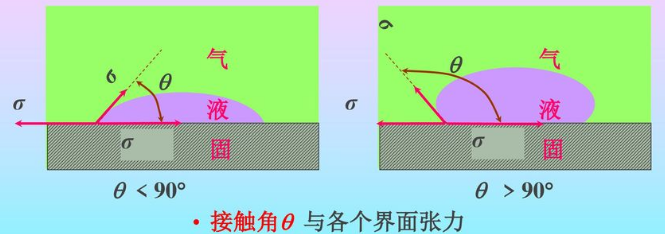$$\cos \theta=\frac{\gamma_{S V}-\gamma_{S L}}{\gamma_{L V}}$$$$\theta \leqslant 180^{\circ}$$沾湿(永远成立，自发过程)，$$\theta \leqslant 90^{\circ}$$浸湿，$$\theta=0^{\circ}$$铺展。固体表面粗糙下接触角更小。参考：《基于特殊润湿性材料的油水分离》

5.相变的热力学分类

spinodal decomposition

6.相变过程的温度条件

7.相变过程的温度条件